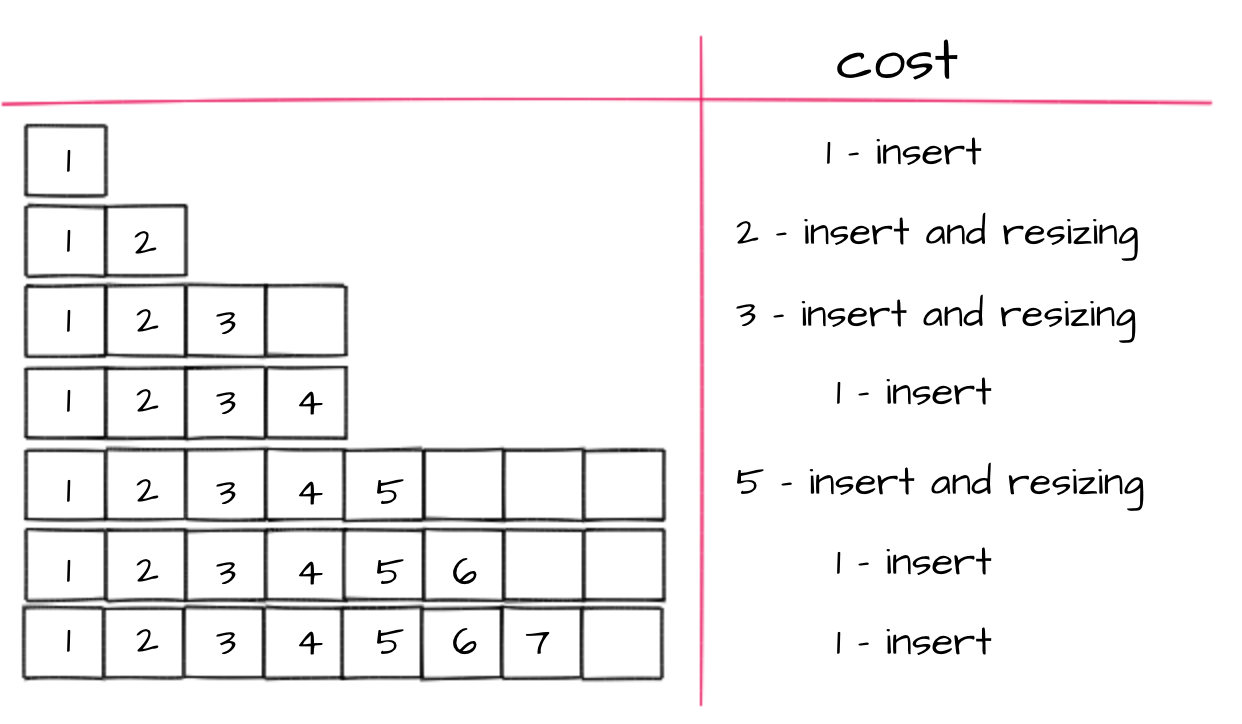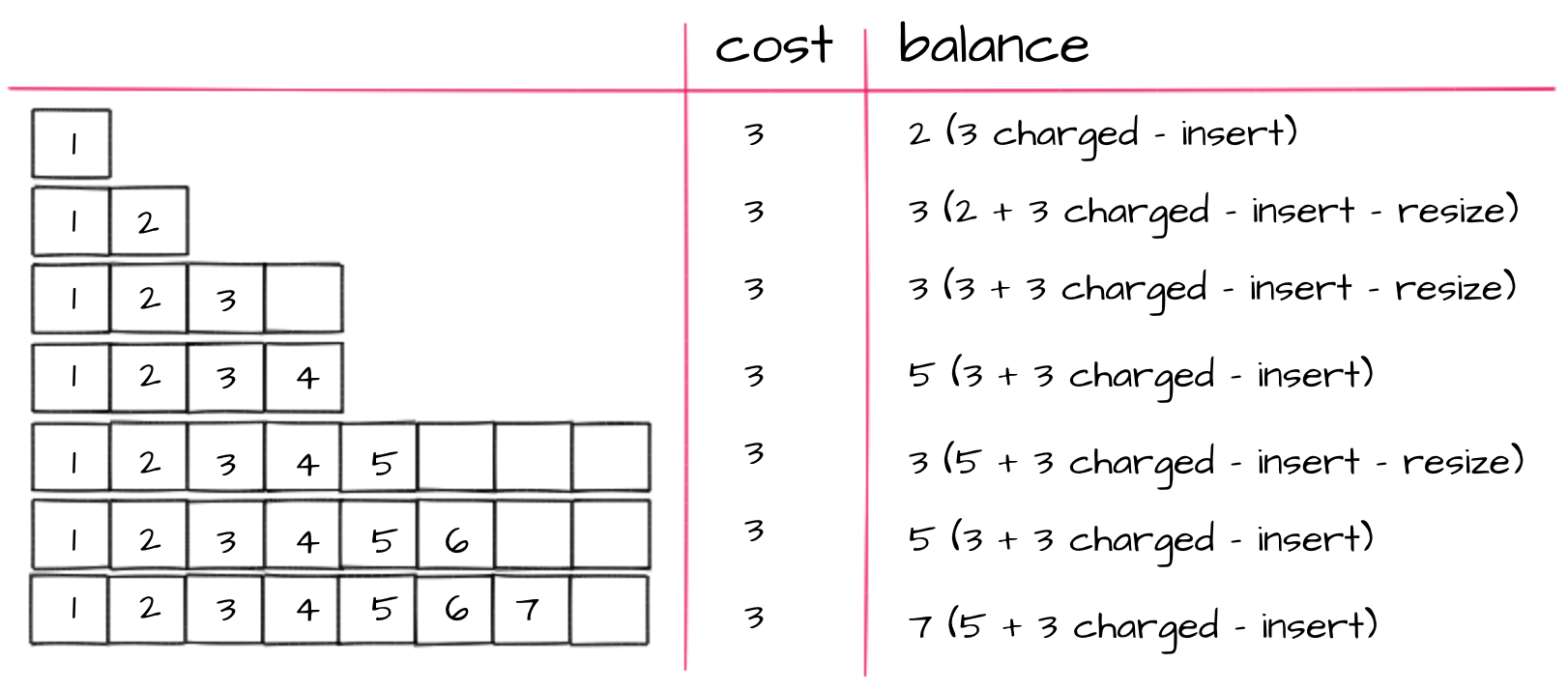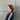# Amortised analysis in the nutshell

Algorithmic complexity is a crucial thing in computer science. Knowing the complexity of algorithms allows to answer following questions:

• How long will a program run on an input?
• How much space will it take?
• Is the problem solvable?

It’s hard to talk about amortised analysis without brushing up on asymptotic analysis first. So, I’m going to bring back some significant stuff about asymptotic analysis and then jump into the main point.

## Asymptotic Analysis

Regular asymptotic analysis looks at the performance of an individual operation asymptotically, as a function of the size of the problem. It is about how the performance of a given operation scales to a large data set.

### Worst-Case and Average-Case Analysis

Worst case analysis always consider a single operation. To find the cost of the algorithm we need to find the worst-case cost of every single operation and then count the number of their executions. If algorithm runs in time T(n) it means that it is and upper bound for any inputs of size n. In fact, the algorithm may take less time on some inputs of that size, because particular operations may cheaper for them, but it doesn’t matter since we counted the worst-cost of every operation in the algorithm.

A worst-cost analysis is not the most reliable way to analyse the algorithms, so there’s an alternative — average-case analysis. In the average-cost analysis we try to calculate the running time for randomly chosen input. It’s kind of harder due to the fact it needs some probabilistic arguments and some assumptions about the inputs distribution, which is not the easiest thing to justify. Despite that it may be a lot more useful, hence the worst-case analysis is often misleading. For example the worst-case running time for the quick-sort algorithm is 2n and the average-case is nlog(n), which in case of large n is a significant difference.

### Order of growth and Big-O notation

Order of growth describes how an algorithm’s time and space complexity is going to increase or decrease when we increase or decrease a size of the input. There are different notations to measure it, but the most popular is Big O notation, which gives the worst-case time complexity. For instance if we have O(f(x)) = g(x) it means that the growth of the function f will never surpass the function g . Let’s consider an example below with the nested for loop. Singe for loop takes n operations so that its time complexity is O(n).

``````for (i = 0; i < n; ++i) {
for (j = 0; j < n; ++j) {
...
}
}``````

This going to take n operations for each value of i due to the nested loop and i has n possible values. So the order of growth is O(n²).

Asymptotic analysis is the most common method for analysing algorithms, but it’s not perfect. Let’s consider an example of two algorithms taking respectively 1000nlog(n) and 2nlog(n) time. In case of asymptotic analysis they are both the same, having asymptotic complexity n*log(n) , so that we can’t judge which one is better as constants are ignored. Another thing is that in asymptotic analysis we always consider input sizes larger that a constant value, but they may be never given as an input, so algorithm which is asymptotically slower, may performs better for the particular situation.

## Amortised Analysis

As it was said earlier, asymptotic analysis is about how the performance of a given operation scales to a large data set. Amortised analysis in the other hand is about how the average of the performance of all of the operations on a large data set scales. Comparing to the average-case analysis, amortised analysis gives an upper bound of the actual cost of an algorithm, which the average-case doesn’t guarantee. To describe it in one sentence we can say that it gives the average performance (over time) of each operation in the worst-case.

When we have some sequence of operations the worst-case doesn’t occur very often in each operation. Operations vary in their costs — some may be cheap and some may be expensive. Let’s take a dynamic array as an example. In the dynamic array the number of elements does not need to be known until program execution and it can be resized at any time. What is important for us is the fact that in the dynamic array only some inserts take a linear time, though others — a constant time. So if the inserts differ in their costs, how we are able to correctly calculate the total time? This is where amortised approach comes into play. It assigns an artificial cost to each operation in the sequence, which is called the amortised cost. It needs the total cost of the algorithm to be bounded by the total number of the amortised costs of all operations. There are three methods used for assigning the amortised cost:

1. Aggregate Method (brute force)
2. Accounting Method (the banker’s method)
3. Potential Method (the physicist’s method)

### Aggregate Method

Let’s take dynamic array as an example. If the array has space available, we simply insert new item in available space. If not following steps are performed:

1. Allocate memory for a larger array of size twice as the old one
2. Copy the contents of old array to new one

Let’s assume first that the cost for insert is equal to 1 unit and resizing an array costs us 1 unit per each element in the array.

What is the time complexity of n insertions using the above scheme?

The cost of i-th insertion is:

``cost_i = if (i − 1 is a power of 2) then i else 1``

And why is that? It’s because whenever the array is full (number of elements already inserted is a power of 2), we need to perform the steps listed above, which costs us array size + 1 , where array size is the cost of coping the table and + 1 is the cost of inserting next element.

Let’s say we want to insert numbers from 1 to 7 to the array, this is how it looks like:So we got the total cost equal to (1 + 2 + 3 + 1 + 5 + 1 + 1) / 7 =~ 2 , so the amortised cost is _O(1) _(reminder: we omit constants).

To sum up, aggregate analysis determines the upper bound T(n) on the total cost of a sequence of n operations, then calculates the amortised cost to be T(n) / n.

### Accounting Method

The idea behind this method is really intuitive. We have an account where we can save up time and every operation is allowed to take some time (it’ our currency) out of the account, but no more than is in there. Many cheap operations help pay for the expensive ones, and if we distribute the cost that way, we can get a better analysis.

Accounting method seeks low-cost operation to be charged a little bit more than its true cost, and the surplus is deposited into the bank account for later use. Expensive operations can then be charged less than their true cost, and the deficit is paid for by the savings in the bank account. In that way we spread the cost of expensive inserts over the entire sequence. The charges to each operation must be set large enough that the balance in the bank account always remains greater than zero, but small enough that no one operation is charged significantly more than its actual cost. Notice that the extra time charged to an operation does not mean that the operation really takes that much time.

Back to the example of the dynamic array. As earlier it costs us 1 unit to insert an element and 1 per each element to be moved to another array. Clearly a charge of 1 unit per insertion is not enough, because there is nothing left over to pay for the moving. Let’s try with charging 3 units per insertion and as earlier we want to insert numbers from 1 to 7 to an empty array:Now whenever an element needs to be moved to the new array, the move is already paid for. The first time an element is moved, when there’s no time in the account, it paid for it with one of its own time units that was charged to it.

### Potential Method

The key to amortised analysis with the potential method is to define the right potential function Φ on states of a data structure with the following properties:

• Φ(h0) = 0, h0 is the initial state of the data structure.
• Φ(ht) ≥ 0

Intuitively, the potential function will keep track of the precharged time at any point in the computation. It measures how much we can by for expensive operations with all the time saved-up earlier. It is analogous to the bank balance in the banker’s method. But interestingly, it depends only on the current state of the data structure. We then define the amortised time of an operation as: c + Φ(h’) − Φ(h), where c is the real cost of the operation, h is the state of data structure before an operation and h’ is the state after operation. Ideally, Φ should be defined so that the amortised time of each operation is small, because the change in potential should be greater than 0 for cheap operations and lower for expensive operations.

For dynamic arrays with resizing by doubling, we can use the potential function:

*Φ(h) = 2n − m,

where n stands for current number of elements in the array and m is the array length. If we start with an array of length 0 and we want to insert the first element we have:

Φ(h0) = 0 – 0 = 0

and for the next elements, we will have: Φ(ht) ≥ 0.

Now we would like become convinced that inserting an element takes amortised constant time. There are two cases:

1. n < m, then the actual cost is 1, n increases by 1, and m does not change. Then the potential increases by 2, so the amortised time is 1 + 2 = 3.
2. If n = m, then the array is doubled, so the actual time is n + 1. But the potential drops from n to 2, so amortised time is n + 1 + (2 − n) = 3.

In both cases, the amortised time is O(1).

## Conclusion

The critical difference between asymptotic and amortised analysis is that the first is dependent on the input itself, while the second is dependent on the sequence of operations the algorithm will execute.

Therefore:

• asymptotic analysis allows us to assert that the complexity of the algorithm when it is given a worst/average case input of size n is bounded by some function f(n)
• amortised analysis allows us to assert that the complexity of the algorithm when it is given an input of unknown characteristics but known size n is no worse than the value of a function f(n)

Published under  on .### Aleksandra

Hi! I'm Aleksandra, a software developer based in Wrocław.